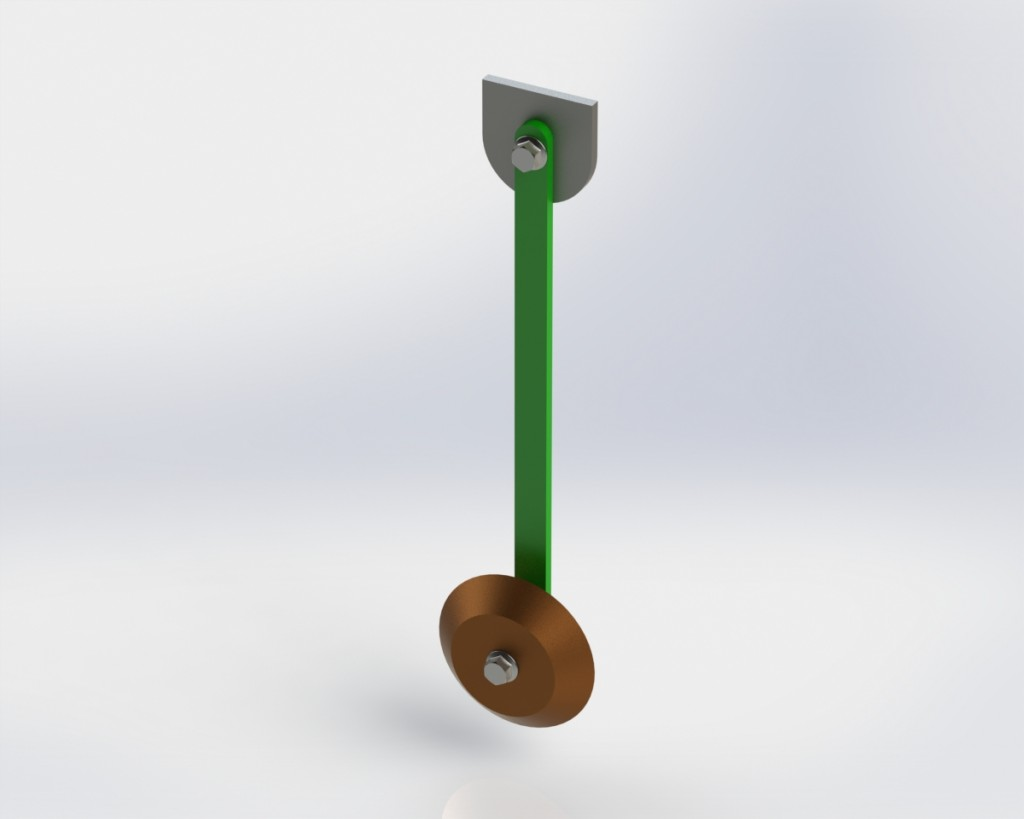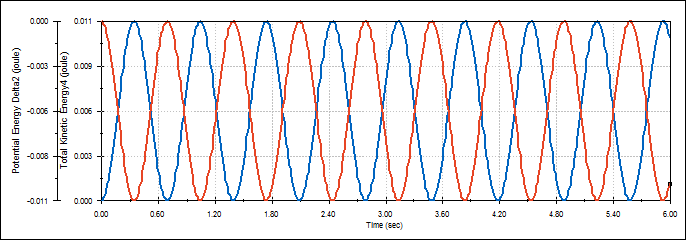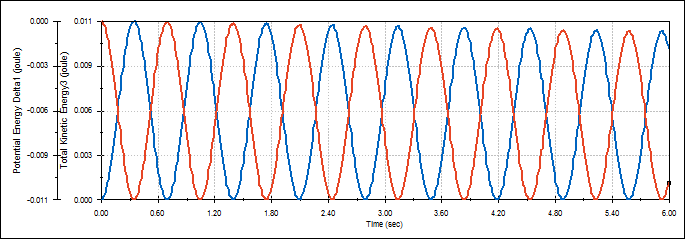Don’t worry, I won’t make you try and remember everything you learned in Physics class and there will NOT be a quiz later! However, hopefully you remember learning about one of the founding principles of physics, the Conservation of Energy. In a nut shell, it states that in the absence of non-conservative forces (such as friction) the total energy of an isolated system remains constant.Take for example a baseball being dropped from your hand. If we make a few assumptions (no air resistance, neglect the balls internal energy (i.e. its temperature)), the energy of the ball remains constant and can be described by its potential energy (a function of its height from the floor) and its kinetic energy (a function of its speed). As the ball is released and just before it begins to fall, all of its energy is potential. As it begins to fall, the potential energy is converted into kinetic energy thanks to gravity. In other words, it accelerates downward, increasing its speed and decreasing its height from the floor. At the instant before it hits the ground, all of its potential energy has been converted to kinetic energy. Got it? Good! So what does all this have to do with SOLIDWORKS?

SOLIDWORKS provides a way to simulate the motion of your models in a virtual world complete with gravity, forces, springs, dampeners, etc, using Motion Analysis. As a quick demonstration of the physics engine in SOLIDWORKS, we’ll use a well known model in physics, the pendulum (model shown above), and show that it adheres to the conservation of energy. Using SOLIDWORKS Motion Analysis, a study was setup such that the top of the rod is fixed to a mount and the only force acting on the model is gravity. When we move the bob to some initial angle off center and start the simulation, it behaves as expected (see below video).

So the pendulum swings, but does it actually obey the conservation of energy? To find out, we can use the plotting tools available in Motion Analysis to show study results, such as force, energy, torque, momentum, etc of chosen components, with respect to time. For our purposes, I’ve created a plot showing the change in kinetic energy and potential energy of the pendulum bob (the weight at the bottom) as a function time.### Potential and Kinetic Energy vs. Time

Based on what we know, the total energy of the system must remain constant with the kinetic and potential energy changing inversely. And this is in fact what the plot shows. Notice as the kinetic energy (blue) is at its maximum, the potential energy (red) is at its minimum.

“Hold up!” you might say… “In the real world there’s always friction.” Well, Motion also allows us to simulate contact between objects and introduce friction (and damping if we so desire). Including friction, however, means our “system” will no longer be truly isolated and that some energy will consequently be lost to the surroundings by heat or sound. When we re-run the simulation, but now with friction, we should see the total energy (kinetic and potential) decrease over time. Let’s take a look at our updated plot.### Potential and Kinetic Energy vs Time (with contact friction)

As expected the total energy of the system decreases with each cycle as seen from the reduced maximums. If allowed to continue, the pendulum would eventually stop moving all together, signifying all its initial energy had been dissipated.

So unless you’re in the business of designing pendulums (or teaching Physics I), this exercise may not seem very useful. It does, however, demonstrate the tools available in SOLIDWORKS Motion, and, most importantly, how understanding the principles of physics and engineering allow us to better interpret and evaluate simulation results. Which hopefully means you’re able to learn something valuable (and accurate) about your models. If you want to learn more about Motion Analysis and some of the other SOLIDWORKS Simulation tools, check out our website for scheduled events, training, and demos. Class dismissed!